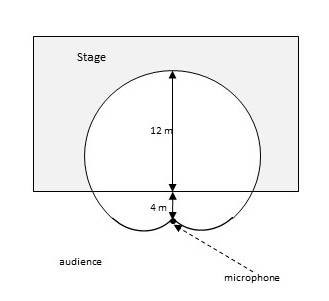# When recording live performances, sound engineers often use a microphone with cardioids' pickup...

## Question:

When recording live performances, sound engineers often use a microphone with cardioids' pickup pattern because it suppresses noise from the audience. Suppose the microphone is placed 4m from the front of the stage (as in the figure below) and the boundary of the optimal pickup region is given by the cardioid {eq}r= 8+8 \sin \theta {/eq}, where r is measured in meters and the microphone is at the pole (origin). The musicians want to know the area they will have on stage within the optimal pickup range of the microphone. Answer their question.## Application of Integrals:

{eq}\\ {/eq}

The area of a cardioid in polar form is given by the formula {eq}\boxed{A=\dfrac{1}{2}\displaystyle \int_{\alpha }^{\beta }r^2d\theta} {/eq}, where {eq}\alpha, \beta {/eq} are the limits of integration and {eq}r=a+a\sin \theta {/eq} represents the equation of a cardioid.

Become a Study.com member to unlock this answer! Create your account

{eq}\\ {/eq}

Given : {eq}r= 8+8 \sin \theta {/eq}

As the sound receiving area covers the entire graph, therefore the limits of {eq}\theta {/eq}...Cardioid in Math: Definition, Equation & Examples

from

Chapter 1 / Lesson 13
34K

This lesson will cover a neat shape studied in upper-level mathematics called a cardioid. We will look at the basic shape, how it is constructed, its equation in polar form, and various examples of these equations and corresponding cardioids.